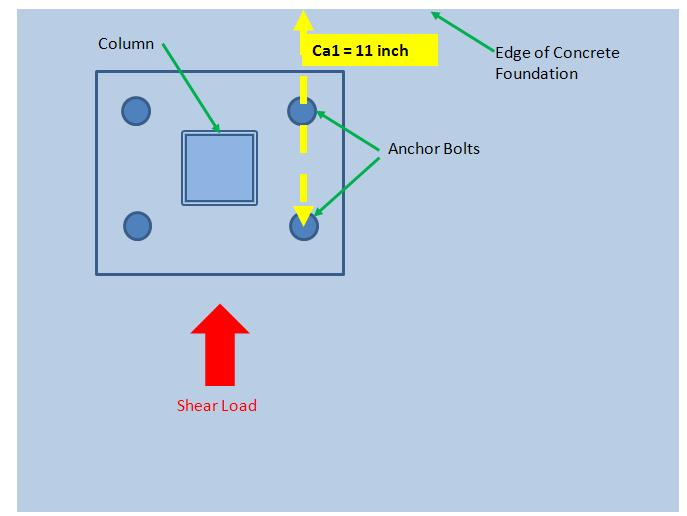# Example of Concrete Anchor Bolt Design Calculation – Part-6: Determining Concrete Breakout Strength of Anchor in Shear

Before diving into the concrete anchor bolt design calculation example for calculating anchor breakout strength in shear, please go through the problem statement and part-1, part-2, part-4 and part-5 of this series. The calculation exercise is carried out according to the ACI 318 appendix D codes.

Calculation

The code gives the following formula for finding out the break out strength in shear for a group of anchor bolts:

φVcbg= φ(AVc/AVcoec,V ψed,V ψc,V ψcp,VVb……………………………..D-22

Where,

Φ – Strength reduction factor for breakout strength and its value for non-reinforcement in tension is 0.70

Avc –  projected concrete failure area of the group of anchors, for calculation of strength in shear (in inch2) as approximated by a rectangle with edges bounded by 1.5*Ca1 (1.5*5 = 7.5 inch in our case) and the free edges of the concrete from the centerline of the anchors. We will calculate it little later.

Ca1 – Distance from the center of an anchor shaft to the edge of concrete in one direction (in inch). Refer Fig.1 below.

Avco – Total projected shear failure area approximated by a square bounded by 1.5*Ca1 (1.5*5 = 7.5 inch in our case) from the centerline of a anchor at all the sides. We will calculate it little later.

ψec,V – Modification factor for the anchor group loaded eccentrically in shear, in our case there is no eccentric loading , so ψec,V=1.

ψed,V – Modification factor for edge effect for the anchor group in shear, we will calculate it later.

Ψc,V – Modification factor based on the presence and absence of crack in the concrete, in our case value of it = 1..

ψcp,V – Modification factor for post weld types of anchor. For cast in place anchor ψcp,V = 1

Vb – Basic concrete break out strength for a single anchor in shear, we will calculate it later.

Calculation of AVcFig.1: Showing Ca1 for calculating Avc and Avco for Anchor Group

Refer the above two figure (Fig.1 and Fig.2), it should be clear by now that

AVC = 16.5*(5+6+16.5) = 453.75 inch2

Calculation of AVco

By referring the above figure (Fig.3), we can calculate

Avco= 16.5*(16.5+16.5) = 544.5 inch2

Calculation of ψed,V

For Ca2 <1.5 Ca1

ψed,V = 0.7+(0.3*Ca2)/(1.5*Ca1) = 0.7+ (0.3*5)/(1.5*11) = 0.79

Where,

Ca2 – Minimum concrete edge distance from anchor perpendicular to Ca1.

Calculation of Vb

Vb=(7*√da*[le/da]0.2 )*λ*√f’c*(Ca1)1.5 ………………………..D-24

Where,

da = Diameter of the anchor bolt in inch., in our case it is 0.75 inch. Remember we have started with the 0.75 inch anchor in the part-1 of this calculation.

le = Length of the anchor inserted inside the concrete in inch. In our case it is 10 inch.

λ = A factor related to the reduced mechanical properties of concrete, for normal weight concrete λ=1.

Ca1 = we already know it from the Fig.1. Its value is 11 inch.

fc’ = Specified compressive strength of the concrete, in our case fc’=4000 psi (refer problem statement of part-1).

So, by putting all the values, the above equation (D-24) becomes

Vb = (7*√0.75*[10/0.75]0.2)*1*(√4000)*111.5

= 23482.02 lb

Now, from the equation D-22, after putting all the values at right hand side,

φVcbg = φ(AVc/AVcoec,V ψed,V ψc,V ψcp,VVb

= 0.7*(453.75/544.5)*1*0.79*1*1*23482.02 lb

= 10821.297 lb

The concrete break out strength in shear for the anchor bolt group according to the ACI codes is calculated as 10821.297 lb. The next part (Part-7) will talk about Concrete Pullout Strength in Shear.

### Shibashis Ghosh

Hi, I am Shibashis, a blogger by passion and engineer by profession. I have written most of the articles for mechGuru.com. For more than a decades i am closely associated with the engineering design/manufacturing simulation technologies.
Disclaimer: I work for Altair. mechGuru.com is my personal blog. Although i have tried to put my neutral opinion while writing about different competitor's technologies, still i would like you to read the articles by keeping my background in mind.

## 2 Replies to “Example of Concrete Anchor Bolt Design Calculation – Part-6: Determining Concrete Breakout Strength of Anchor in Shear”

1.asad says:

nyc job

2.asad says:

bt i guess there is some prob with the area calculated for shear for group of anchor..
there should be 35 degree leverage to the edge…

This site uses Akismet to reduce spam. Learn how your comment data is processed.